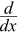# Product Rule

The Derivative tells us the slope of a function at any point.

## Product Rule

The product rule tells us how to differentiate the product of two functions:

(fg)’ = fg’ + gf’

Note: the little mark means "Derivative of", and f and g are functions.

### Example: What is the derivative of cos(x)sin(x) ?

The Product Rule says:

(fg)’ = f g’ + f’ g

In our case:

• f = cos
• g = sin

We know (from Derivative Rules):

•cos(x) = −sin(x)
•sin(x) = cos(x)

So:

the derivative of cos(x)sin(x) = cos(x)cos(x) − sin(x)sin(x)

= cos2(x) − sin2(x)

## Why Does It Work?

When we multiply two functions f(x) and g(x) the result is the area fg:When we increase x a little, both f and g will change a little also (by Δf and Δg). In this case they both increase making the area bigger.

How much bigger?

Increase in area = fΔg + ΔfΔg + gΔf

As the change in x heads towards zero, the "ΔfΔg" term also heads to zero, and we get:

(fg)’ = fg’ + gf’

## Alternative Notation

An alternative way of writing it (called Leibniz Notation) is:

ddx(uv) = dudxv + udvdx

## Three Functions

For three functions multiplied together we get this:

(fgh)’ =  f’gh + fg’h  + fgh’